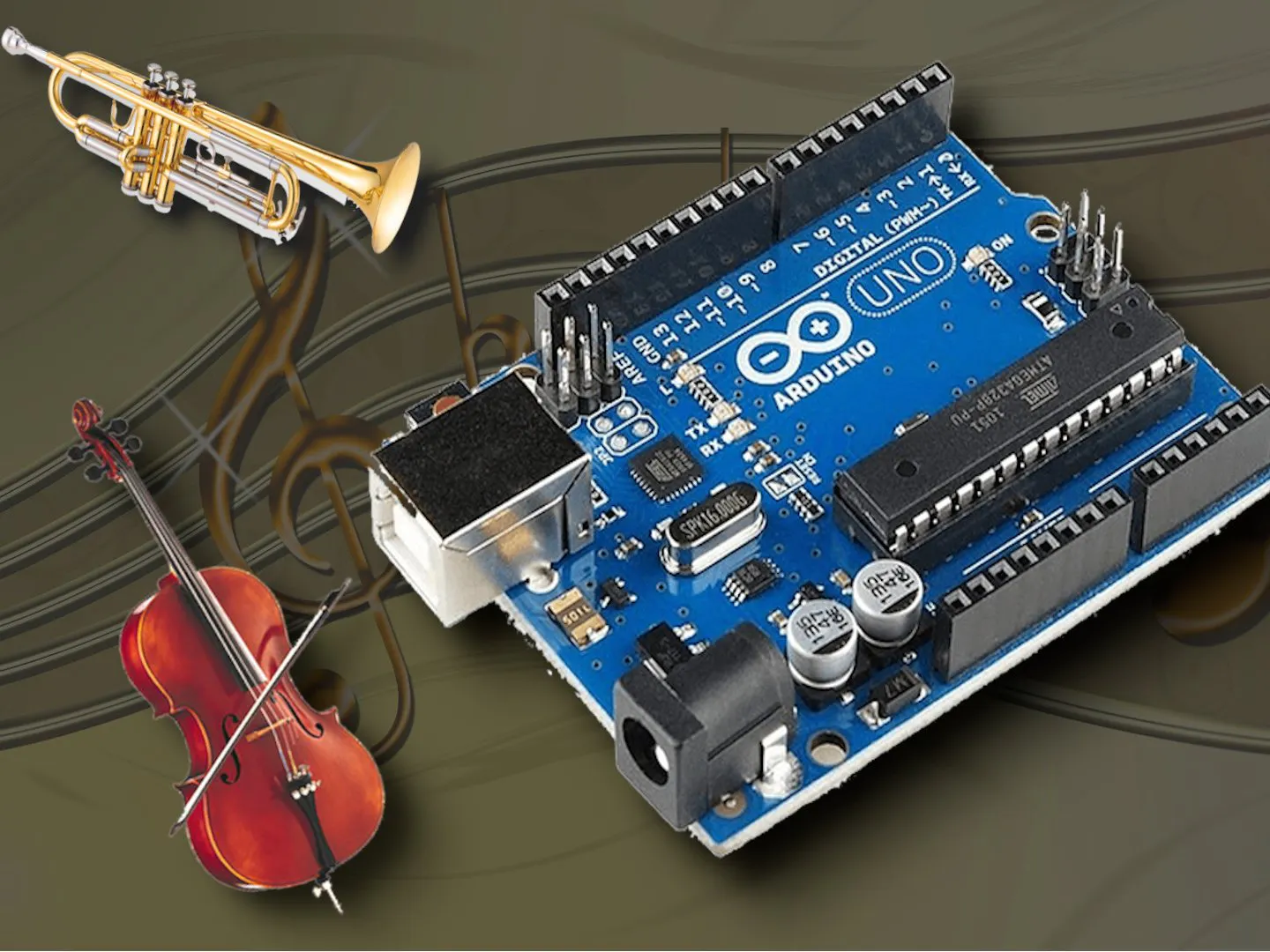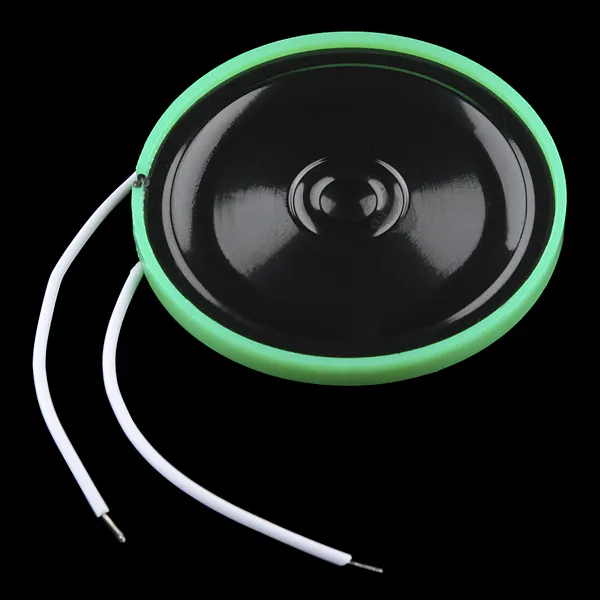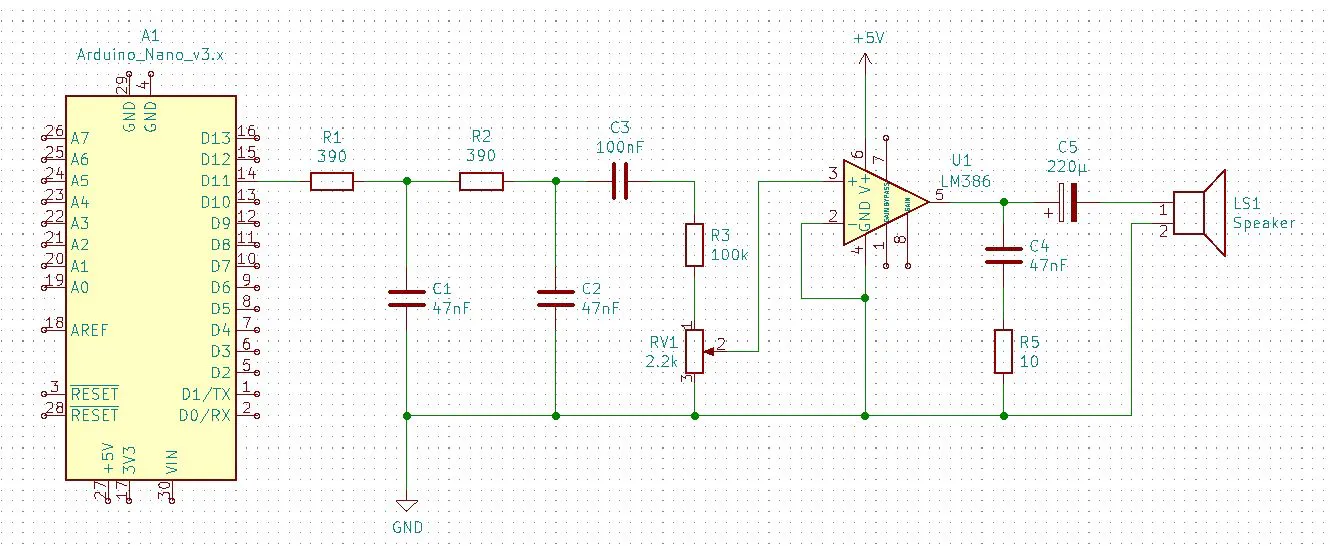# Arduino as Waveform Synthesizer for Music

Imitate musical instruments and make music with an Arduino and only few external components!

BeginnerProtip2 hours14,989## Things used in this project

### Hardware componentsArduino Nano R3
×1Speaker: 0.25W, 8 ohms
×1×1
 Resistors, Capacitors (Generic)
×1

### Software apps and online servicesMicrosoft Visual Studio Code Extension for Arduino

## Schematics

### Schematic## Code

### chimes.ino

C/C++
Demo shows how to use it
```/*This work is licensed under the Creative Commons Attribution-ShareAlike 4.0 International License.

#include "chimes.h"
using namespace Chimes;
//Sum of ADSR values must not exceed 100%
uint8_t envelope[] = {
0,  //attack[%]
20, //decay[%]
0,  //sustain[%]
80, //release[%]
16  //Sustain Level 1..32
};

void setup()
{
init(
TRI, //TRI: Triangle, RECT: Rectangle
50,  //duty cycle 0..100%, only matters for Triangle and Rectangle
envelope);
}

uint16_t melody[] = {{330, 1000}, {415, 1000}, {370, 1000}, {247, 1000}, {0, 1000}, {330, 1000}, {370, 1000}, {415, 1000}, {330, 1000}, {0, 1000}, {415, 1000}, {330, 1000}, {370, 1000}, {247, 1000}, {0, 1000}, {247, 1000}, {370, 1000}, {415, 1000}, {330, 1000}};

void loop()
{
static int i = 0;
if (i < 19 && !isPlaying())
{
play(melody[i], melody[i]);
i++;
}
}
```

### chimes.h

C/C++
Include file
```/*This work is licensed under the Creative Commons Attribution-ShareAlike 4.0 International License.

#ifndef CHIMES_H
#define CHIMES_H
#include "Arduino.h"

enum waveform
{
SINE, //Sinus
RECT, //Triangle
TRI,  //Rectangle
PAUSE //Internal, do not use
};
#define MAX_VOLUME 32

namespace Chimes
{
void init(uint8_t waveform = SINE, uint8_t duty_cycle = 50, uint8_t *envelope = NULL);
void play(uint16_t freq, uint16_t duration);

//Returns true while note is playing
boolean isPlaying();
} // namespace Chimes

#endif
```

### chimes.cpp

C/C++
```/*This work is licensed under the Creative Commons Attribution-ShareAlike 4.0 International License.

#include <Math.h>
#include "chimes.h"

#define ISR_CYCLE 16 //16s

char strbuf;
uint16_t ADSR_default[] = {0, 0, 100, 0, MAX_VOLUME};
uint16_t nSamples; //Number of samples in Array
uint32_t tPeriod;
uint8_t *samples; //Array with samples
uint8_t *_envelope, _waveform, _duty_cycle;

{
ATTACK,
DECAY,
SUSTAIN,
RELEASE
};

namespace Chimes
{
void init(uint8_t waveform, uint8_t duty_cycle, uint8_t *envelope)
{
Serial.begin(115200);
//PWM Signal generation
DDRB |= (1 << PB3) + (1 << PB0);				  //OC2A, Pin 11
TCCR2A = (1 << WGM21) + (1 << WGM20);			  //Fast PWM
TCCR2A |= (0 << COM2A0) + (1 << COM2A1);		  //Set OC2A on compare match, clear OC2A at BOTTOM,(inverting mode).
TCCR2B = (0 << CS22) + (0 << CS21) + (1 << CS20); //No Prescaling
samples = (uint8_t *)malloc(0);
_waveform = waveform;
_duty_cycle = duty_cycle;
_envelope = envelope;
}

void play(uint16_t freq, uint16_t duration)
{
uint8_t waveform = _waveform;
//Init adsr according to the length of the note
for (int i = 0; i < 4; i++)
{
if (_envelope)
{
ADSR_env[i] = (uint32_t)_envelope[i] * duration / 100;
}
else
{
}
}
ADSR_env = _envelope ? _envelope : MAX_VOLUME;

if (freq == 0)
{ //Pause
tPeriod = ISR_CYCLE * 100;
waveform = PAUSE;
}
else
tPeriod = 1E6 / freq;

nSamples = tPeriod / ISR_CYCLE;
realloc(samples, nSamples);
uint16_t nDuty = (_duty_cycle * nSamples) / 100;

switch (waveform)
{
case SINE: //Sinewave
for (int i = 0; i < nSamples; i++)
{
samples[i] = 128 + 127 * sin(2 * PI * i / nSamples);
}
break;

case TRI: //Triangle
for (int16_t i = 0; i < nSamples; i++)
{
if (i < nDuty)
{
samples[i] = 255 * (double)i / nDuty; //Rise
}
else
{
samples[i] = 255 * (1 - (double)(i - nDuty) / (nSamples - nDuty)); //Fall
}
}
break;
case RECT: //Rectangle
for (int16_t i = 0; i < nSamples; i++)
{
i < nDuty ? samples[i] = 255 : samples[i] = 0;
}
break;
case PAUSE: //Rectangle
memset(samples, 0, nSamples);
}
TIMSK2 = (1 << TOIE2);
/*for(uint16_t i = 0; i < nSamples; i++) {
sprintf(strbuf, "%d: %d", i, samples[i]);
Serial.println(strbuf);
}*/
}

//Returns true, while note is playing
boolean isPlaying()
{
return (1 << TOIE2) & TIMSK2;
}
} // namespace Chimes

//Called every 16s, when TIMER1 overflows
ISR(TIMER2_OVF_vect)
{
static uint16_t cnt; //Index counter
static uint8_t sustain_lvl, vol;

//Set OCR2A to the next value in sample array, this will change the duty cycle accordingly
OCR2A = vol * samples[cnt] / MAX_VOLUME;
if (cnt < nSamples - 1)
{
cnt++;
}
else
{
cnt = 0;
{ //every 10 millisecond

{
case ATTACK:
{
if (vol == MAX_VOLUME)
{ //Attack phase over
}
}
else
{
vol = MAX_VOLUME;
}
break;

case DECAY:
{
sustain_lvl = _sustain_lvl;
if (vol <= sustain_lvl)
{
}
}
else
{
sustain_lvl = MAX_VOLUME;
}
break;

case SUSTAIN:
{
}

break;
case RELEASE:
{
if (vol == 0)
{ //Attack phase over
TIMSK2 = (0 << TOIE2);
}
}
else
{
vol = 0;